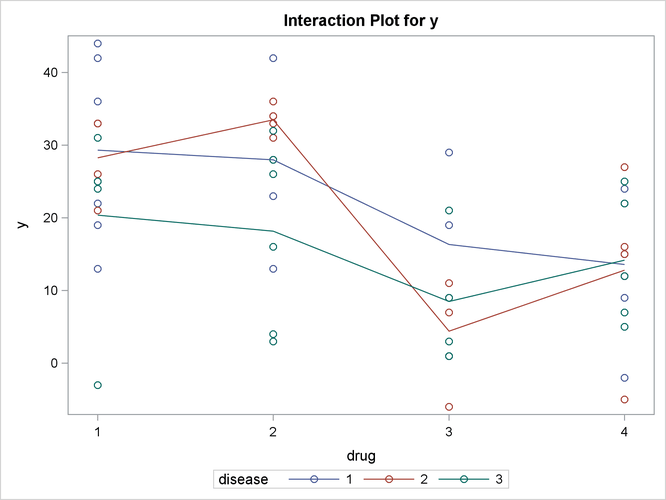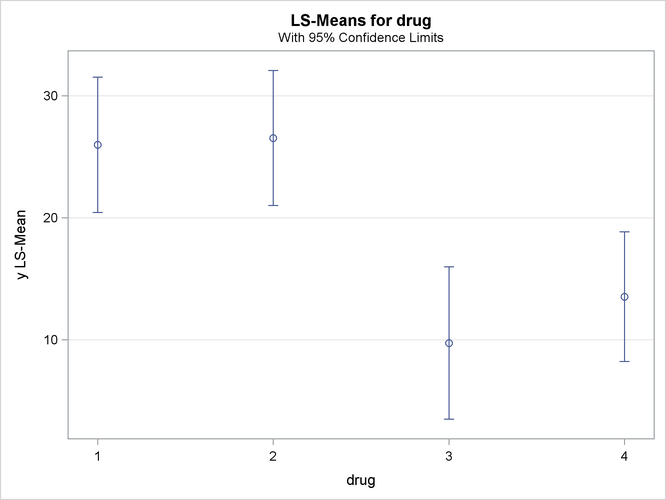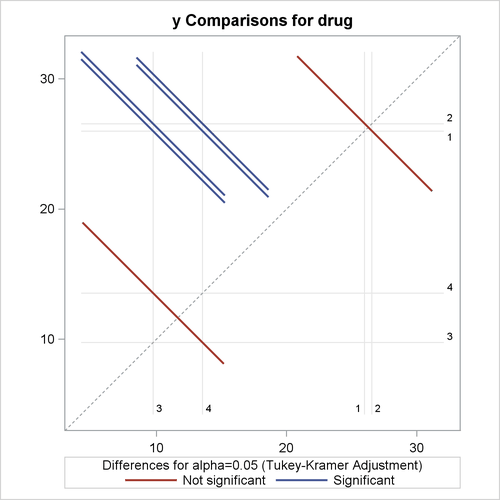Example 42.3 Unbalanced ANOVA for Two-Way Design with Interaction

This example uses data from Kutner (1974, p. 98) to illustrate a two-way analysis of variance. The original data source is Afifi and Azen (1972, p. 166). These statements produce Output 42.3.1 and Output 42.3.2.

title 'Unbalanced Two-Way Analysis of Variance';
data a;
input drug disease @;
do i=1 to 6;
input y @;
output;
end;
datalines;
1 1 42 44 36 13 19 22
1 2 33  . 26  . 33 21
1 3 31 -3  . 25 25 24
2 1 28  . 23 34 42 13
2 2  . 34 33 31  . 36
2 3  3 26 28 32  4 16
3 1  .  .  1 29  . 19
3 2  . 11  9  7  1 -6
3 3 21  1  .  9  3  .
4 1 24  .  9 22 -2 15
4 2 27 12 12 -5 16 15
4 3 22  7 25  5 12  .
;
proc glm;
class drug disease;
model y=drug disease drug*disease / ss1 ss2 ss3 ss4;
run;

Output 42.3.1: Classes and Levels for Unbalanced Two-Way Design

 Unbalanced Two-Way Analysis of Variance

The GLM Procedure

Class Level Information
Class Levels Values
drug 4 1 2 3 4
disease 3 1 2 3

 Number of Observations Read 72 58

Output 42.3.2: Analysis of Variance for Unbalanced Two-Way Design

 Unbalanced Two-Way Analysis of Variance

The GLM Procedure

Dependent Variable: y

Source DF Sum of Squares Mean Square F Value Pr > F
Model 11 4259.338506 387.212591 3.51 0.0013
Error 46 5080.816667 110.452536
Corrected Total 57 9340.155172

R-Square Coeff Var Root MSE y Mean
0.456024 55.66750 10.50964 18.87931

Source DF Type I SS Mean Square F Value Pr > F
drug 3 3133.238506 1044.412835 9.46 <.0001
disease 2 418.833741 209.416870 1.90 0.1617
drug*disease 6 707.266259 117.877710 1.07 0.3958

Source DF Type II SS Mean Square F Value Pr > F
drug 3 3063.432863 1021.144288 9.25 <.0001
disease 2 418.833741 209.416870 1.90 0.1617
drug*disease 6 707.266259 117.877710 1.07 0.3958

Source DF Type III SS Mean Square F Value Pr > F
drug 3 2997.471860 999.157287 9.05 <.0001
disease 2 415.873046 207.936523 1.88 0.1637
drug*disease 6 707.266259 117.877710 1.07 0.3958

Source DF Type IV SS Mean Square F Value Pr > F
drug 3 2997.471860 999.157287 9.05 <.0001
disease 2 415.873046 207.936523 1.88 0.1637
drug*disease 6 707.266259 117.877710 1.07 0.3958

Note the differences among the four types of sums of squares. The Type I sum of squares for drug essentially tests for differences between the expected values of the arithmetic mean response for different drugs, unadjusted for the effect of disease. By contrast, the Type II sum of squares for drug measures the differences between arithmetic means for each drug after adjusting for disease. The Type III sum of squares measures the differences between predicted drug means over a balanced drugdisease population—that is, between the LS-means for drug. Finally, the Type IV sum of squares is the same as the Type III sum of squares in this case, since there are data for every drug-by-disease combination.

No matter which sum of squares you prefer to use, this analysis shows a significant difference among the four drugs, while the disease effect and the drug-by-disease interaction are not significant. As the previous discussion indicates, Type III sums of squares correspond to differences between LS-means, so you can follow up the Type III tests with a multiple-comparison analysis of the drug LS-means. Since the GLM procedure is interactive, you can accomplish this by submitting the following statements after the previous ones that performed the ANOVA.

run;

Both the LS-means themselves and a matrix of adjusted p-values for pairwise differences between them are displayed; see Output 42.3.3 and Output 42.3.4.

Output 42.3.3: LS-Means for Unbalanced ANOVA

 Unbalanced Two-Way Analysis of Variance

The GLM Procedure
Least Squares Means

drug y LSMEAN LSMEAN Number
1 25.9944444 1
2 26.5555556 2
3 9.7444444 3
4 13.5444444 4

Output 42.3.4: Adjusted p-Values for Pairwise LS-Mean Differences

Least Squares Means for effect drug
Pr > |t| for H0: LSMean(i)=LSMean(j)
Dependent Variable: y
i/j 1 2 3 4
1   0.9989 0.0016 0.0107
2 0.9989   0.0011 0.0071
3 0.0016 0.0011   0.7870
4 0.0107 0.0071 0.7870

The multiple-comparison analysis shows that drugs 1 and 2 have very similar effects, and that drugs 3 and 4 are also insignificantly different from each other. Evidently, the main contribution to the significant drug effect is the difference between the 1/2 pair and the 3/4 pair.

If ODS Graphics is enabled for the previous analysis, GLM also displays three additional plots by default:

• an interaction plot for the effects of disease and drug

• a mean plot of the drug LS-means

• a plot of the adjusted pairwise differences and their significance levels

The following statements reproduce the previous analysis with ODS Graphics enabled. Additionally, the PLOTS=MEANPLOT(CL) option specifies that confidence limits for the LS-means should also be displayed in the mean plot. The graphical results are shown in Output 42.3.5 through Output 42.3.7.

ods graphics on;
proc glm plot=meanplot(cl);
class drug disease;
model y=drug disease drug*disease;
run;
ods graphics off;

Output 42.3.5: Plot of Response by Drug and DiseaseOutput 42.3.6: Plot of Response LS-Means for DrugOutput 42.3.7: Plot of Response LS-Mean Differences for DrugThe significance of the drug differences is difficult to discern in the original data, as displayed in Output 42.3.5, but the plot of just the LS-means and their individual confidence limits in Output 42.3.6 makes it clearer. Finally, Output 42.3.7 indicates conclusively that the significance of the effect of drug is due to the difference between the two drug pairs (1, 2) and (3, 4).